# JP4183576B2 - Scanning surface shape measuring apparatus and surface shape measuring method - Google Patents

Scanning surface shape measuring apparatus and surface shape measuring method Download PDF

## Info

Publication number
JP4183576B2
JP4183576B2 JP2003206673A JP2003206673A JP4183576B2 JP 4183576 B2 JP4183576 B2 JP 4183576B2 JP 2003206673 A JP2003206673 A JP 2003206673A JP 2003206673 A JP2003206673 A JP 2003206673A JP 4183576 B2 JP4183576 B2 JP 4183576B2
Authority
JP
Japan
Prior art keywords
surface shape
surface
measurement
test surface
shape
Prior art date
Legal status (The legal status is an assumption and is not a legal conclusion. Google has not performed a legal analysis and makes no representation as to the accuracy of the status listed.)
Active
Application number
JP2003206673A
Other languages
Japanese (ja)
Other versions
JP2005055221A (en
Inventor

Original Assignee

Priority date (The priority date is an assumption and is not a legal conclusion. Google has not performed a legal analysis and makes no representation as to the accuracy of the date listed.)
Filing date
Publication date
Application filed by 株式会社ミツトヨ filed Critical 株式会社ミツトヨ
Priority to JP2003206673A priority Critical patent/JP4183576B2/en
Publication of JP2005055221A publication Critical patent/JP2005055221A/en
Application granted granted Critical
Publication of JP4183576B2 publication Critical patent/JP4183576B2/en
Application status is Active legal-status Critical
Anticipated expiration legal-status Critical

## Images

••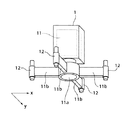•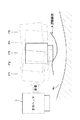•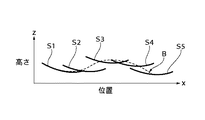•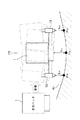•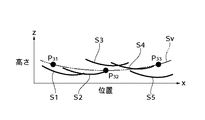•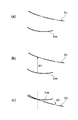•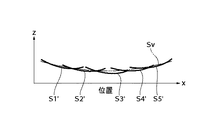•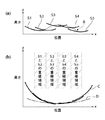•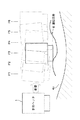•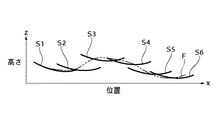•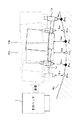•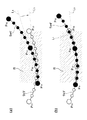## Description


BACKGROUND OF THE INVENTION
The present invention relates to a scanning surface shape measuring apparatus and a surface shape measuring method for measuring the shape of a test surface by scanning the test surface with an interferometer.

[Prior art]
One apparatus for measuring the planar shape with high accuracy is an interferometer. The interferometer generates interference fringes from the reflected light from the reference surface and the reflected light from the test surface, and measures the shape of the test surface based on the interference fringes, and observes the laser light. The optical system projects an enlarged image onto an area, the optical system forms an image of interference fringes generated during measurement, and an image sensor such as a CCD that converts the formed interference fringe image into an electrical signal. The observation area and lateral resolution of the interferometer are determined by the optical design and the number of pixels of the image sensor. In the interferometer, when the observation area is increased, the lateral resolution is lowered, and conversely, when the lateral resolution is increased, the observation area is reduced. Therefore, it is necessary to individually select the interferometer according to the size of the surface to be measured and the lateral resolution at the time of measurement. Furthermore, in an interferometer having a large observation area, since the optical parts to be used are large, the apparatus size tends to be large and the price tends to be expensive.

As a method of measuring the shape of a large test surface using an interferometer having a small observation area, there is a method of measuring the shape of the test surface by scanning the interferometer with respect to the test surface. In the case of this method, in order to perform highly accurate surface shape measurement on the surface to be measured, it is required to perform ideal scanning so that the motion error during scanning is extremely small. In addition, as described in the Journal of Precision Engineering Vol.61, No.12,1995 “Study on Ultra-Smooth Polishing Technology for Aspherical Optical Elements (1st Report) -Development of On-Machine Shape Measuring Device-” There is a method of correcting and correcting the motion error with high accuracy.

Further, in the measurement method of measuring the test surface shape by scanning the test surface with the interferometer, a method for measuring the test surface shape without being affected by the movement error at the time of scanning, It is proposed in “Measurement of large plane surface shape by connecting small-aperture interferograms” (Optical engineering Feb., 1994, Vol.33, No.2, (608)). This method calculates the shape of the entire test surface by scanning the interferometer while overlapping the observation region with respect to the test surface, and connecting the shape of each region obtained from each observation region. is there. Here, when connecting the calculated shapes of the adjacent regions, the slope component of the shape of each region is corrected so that the data of the overlapping regions in the shape of each region match. However, this method requires sufficiently high measurement accuracy for each observation region.

[Non-Patent Document 1]
Journal of Japan Society for Precision Engineering Vol.61, No.12,1995 "Study on ultra-smooth polishing technology for aspherical optical elements (1st report) -Development of on-machine shape measuring device-"
[Non-Patent Document 2]
“Measurement of large plane surface shape by connecting small-aperture interferograms” (Optical engineering Feb., 1994, Vol.33, No.2, (608))

[Problems to be solved by the invention]
However, in the case of the measurement method in which the interferometer is scanned with respect to the above-described test surface, it is possible to realize a scanning mechanism capable of performing an ideal scan so that a motion error during scanning becomes very small. In addition, in the case of a method for correcting and correcting the motion error with high accuracy in advance, it is very difficult to correct the motion error during scanning with high accuracy.

In addition, in the case of a method for measuring the shape of the surface to be measured without being affected by motion errors, a sufficiently high measurement accuracy is required for each observation region, but it is possible to achieve the measurement accuracy as required. At present, it is difficult, and there is an error larger than the error corresponding to the required measurement accuracy in the shape calculated from each observation region. As a result, when connecting the calculated shape of each region, each error is accumulated and becomes very large, and it is difficult to obtain the shape of the test surface with sufficiently high measurement accuracy. Conceivable.

The present invention has been made to solve the above-described problems, and reduces the influence of motion error during scanning, and has a highly accurate surface shape with respect to a large test surface using an interferometer with a small observation area. An object of the present invention is to provide a scanning surface shape measuring apparatus and a surface shape measuring method capable of performing measurement.

[Means for Solving the Problems]
In order to achieve the above object, the present invention provides a partial surface shape corresponding to the observation region based on interference fringes generated by reflected light from each of the partial surface corresponding to the observation region on the surface to be measured and the reference surface. A distance detecting means for detecting a distance in the height direction between the interferometer to be measured and at least one position on the test surface; and a mounting portion for mounting the interferometer and the distance detecting means. Scanning means for moving the mounting portion relative to the surface to be measured so that the observation region of the interferometer is at least in contact with adjacent measurement positions in order to a plurality of measurement positions; and Among them, the distance in the height direction between the at least one measurement position detected by the distance detection means and the at least one position on the surface to be measured and the at least one measurement position measured by the interferometer Partial surface shape A virtual test surface shape based on the calculated virtual test surface shape, and connecting the partial surface shapes measured at the plurality of measurement positions with reference to the calculated virtual test surface shape. And a surface shape calculating means for calculating the shape.

Further, the surface shape calculating means corrects each of the partial surface shapes so that a residual with the corresponding portion of the virtual test surface shape is minimized, and connects the corrected partial surface shapes respectively. It is characterized by.

Further, the distance detection means detects distances in the height direction between a plurality of different positions on the test surface, and the surface shape calculation means calculates the virtual test surface shape by calculating the virtual test surface shape. A distance in the height direction between a plurality of different positions on the surface to be detected, each detected by a distance detecting means, is used.

Further, the distance detecting means detects a distance in the height direction between at least one position outside the observation region on the surface to be measured.

In order to achieve the above object, the present invention provides a partial surface corresponding to the observation region based on interference fringes generated by reflected light from each of the partial surface corresponding to the observation region on the surface to be measured and the reference surface. A mounting portion on which a distance detecting means for detecting a distance in the height direction between the interferometer for measuring the shape and at least one position on the surface to be measured is mounted in order to a plurality of measurement positions. A surface shape measuring method for measuring a surface shape of the test surface by moving the relative position with respect to the test surface so that the observation region of the interferometer is at least in contact with the measurement position, Among the measurement positions, the distance in the height direction between at least one measurement position detected by the distance detection means and the at least one position on the surface to be measured and the interferometer at the at least one measurement position Measured by By calculating the virtual test surface shape based on the calculated partial surface shape, by connecting the partial surface shapes respectively measured at the plurality of measurement positions with reference to the calculated virtual test surface shape, The shape of the test surface is calculated.

DETAILED DESCRIPTION OF THE INVENTION
Hereinafter, embodiments of the present invention will be described with reference to the drawings.

(First embodiment)
FIG. 1 is a diagram schematically showing the configuration of a scanning surface shape measuring apparatus according to a first embodiment of the present invention. FIG. 2 is a view around an opening 11a of an interferometer 11 built in the measuring head 1 of FIG. FIG.

As shown in FIG. 1, the planar shape measuring apparatus includes an optical surface plate 5 having an optical support surface 5a formed on the upper surface, and an inspection of an inspection object 4 placed on the optical support surface 5a of the optical surface plate 5. The measurement head 1 that outputs data for obtaining the surface shape of the surface 4a, and the measurement head 1 is scanned with the optical support surface 5a of the optical surface plate 5 so that the measurement head 1 scans the inspection surface 4a of the inspection object 4. And a scanning mechanism 2 for moving in parallel.

As shown in FIG. 2, the measuring head 1 uses, as image data, optical interference fringe images generated by reflected light from a reference surface (not shown) and a partial surface corresponding to an observation region on the surface 4a. The interferometer 11 for converting and outputting is incorporated. This image data is data indicating a partial surface shape corresponding to the observation region. The interferometer 11 is formed with an opening 11a for capturing reflected light from the test surface 4a, and a plurality of arms 11b projecting in the horizontal direction are provided around the opening 11a. . A distance sensor 12 is attached to the tip of each arm 11b.

In the present embodiment, four arms 11b are provided at equiangular intervals around the opening 11a of the interferometer 11, and the two arms 11b face each other in the x direction with the opening 11a interposed therebetween. Are arranged to be. The remaining two arms 11b are arranged so as to face each other in the y direction across the opening 11a. That is, in the present embodiment, four distance sensors 12 are provided, and among the distance sensors 12, two distance sensors 12 are opposed to each other along the x direction with the opening 11a interposed therebetween. Has been placed. The remaining two distance sensors 12 are arranged so as to face each other along the y direction with the opening 11a interposed therebetween. The number of arms 11b and distance sensors 12 and their arrangement are not limited to the above, and any number and any arrangement can be used.

Each distance sensor 12 is a sensor for measuring the vertical height from the position of each distance sensor 12 to the test surface 4a. As each distance sensor 12, a general uniaxial distance sensor such as a capacitance sensor or an optical sensor can be used. Here, as the optical sensor, a sensor using a focal position detection method or the like is used. The output of each distance sensor 12 is output after being converted into height data indicating the vertical height to the test surface 4a in the measuring head 1.

As shown in FIG. 1, the scanning mechanism 2 has a gate-shaped support portion 3, and this support portion 3 has a drive source (not shown) and is provided with a guide rail 2 b provided on the optical surface plate 5. It is configured to be able to run in the y direction while being guided by the vehicle. The support portion 3 is provided with a guide rail 2a extending in the x direction orthogonal to the y direction, and a movable table 2c is movably supported by the guide rail 2a. The movable table 2c has a drive source (not shown), and is configured to be capable of traveling in the x direction while being guided by the guide rail 2a by the drive source. The measurement head 1 can be scanned in the y direction with respect to the test surface 4a by the movement of the support portion 3 of the scanning mechanism 2, and the measurement head 1 can be scanned in the x direction with respect to the test surface 4a by the movement of the movable table 2c. Can be scanned.

The image data and height data output from the measuring head 1 are input to the image processing control device 6 via the cable 6a. The image processing control device 6 is configured by a device such as a computer including a CPU, a memory, an interface, and the like. The image processing control device 6 analyzes the input data and performs a surface shape calculation process for calculating the surface shape to be examined. Details of the surface shape calculation process will be described later. Further, the image processing control device 6 performs scanning control for scanning the measuring head 1. In this scanning control, specifically, a scanning control signal for instructing a moving speed, a moving direction, a moving position, etc. with respect to each of the moving table 2c and the supporting unit 3 is generated. 8 is input. Further, a monitor 7 is connected to the image processing control device 6, and the monitor 7 selectively displays set measurement conditions, image data (interference fringes), calculated surface shape to be measured, and the like. Is possible.

The scanning drive unit 8 generates a driving signal for the movable table 2 c and a driving signal for the support unit 3 based on each scanning control signal input from the image processing control unit 6. The driving signal for the moving table 2c is output to the moving table 2c via the signal line 8a, and the driving signal for the supporting unit 3 is output to the supporting unit 3 via the signal line 8b.

In the present embodiment, an example will be described in which the measurement head 1 is scanned so that the measurement head 1 sequentially moves to each preset measurement position in the x direction. Here, each measurement position is determined so that a part of the observation area by the interferometer 11 overlaps or the observation area is in contact with each other at the measurement positions adjacent to each other. That is, the measurement positions adjacent to each other are determined such that the interval is smaller than or equal to the length of the observation region of the interferometer 11 in the x direction. Further, the number of measurement positions is determined according to the size of the observation region by the interferometer 11 and the size of the measurement target region of the test surface 4a.

The image processing control device 6 calculates the partial surface shape of the test surface 4a at each measurement position based on the image data obtained by the interferometer 11 for each measurement position, and at least one of the measurement positions. The virtual test surface shape is calculated based on the height data obtained by the distance sensor 12 at the measurement position and the image data obtained by the interferometer 11 at the at least one measurement position. Then, the surface shape of the test surface 4a is calculated by connecting the partial surface shapes at the respective measurement positions with reference to the calculated virtual test surface shape. Here, in the measurement positions adjacent to each other, a part of the observation region by the interferometer 11 overlaps or the observation region touches, so that the partial surface shapes adjacent to each other have or touch the overlap region.

When connecting the partial surface shapes obtained at each of the measurement positions, each partial surface shape is set so that the residual between each partial surface shape and the corresponding portion of the virtual test surface shape is minimized. Thus, operations such as movement and rotation are performed, and the partial surface shapes after the operation are connected.

Next, the test surface shape calculation process will be specifically described with reference to FIGS. FIG. 3 is a diagram schematically showing an example of the measurement position and the movement error when the measurement head 1 of FIG. 1 is scanned in the x direction, and FIG. 4 is each measurement position obtained by the x direction scan of the measurement head 1 of FIG. FIG. 5 is a diagram schematically showing an example of the partial surface shape and the amount of error added to the partial surface shape due to the movement error associated with the scanning of the measuring head 1, and FIG. 5 is the height data used for calculating the virtual test surface shape FIG. 6 schematically shows an example of the calculated virtual test surface shape, and FIG. 7 corrects the partial surface shape based on the virtual test surface shape of FIG. FIG. 8 is a diagram schematically showing each partial surface shape corrected based on the virtual test surface shape in FIG. 6.

In the present embodiment, as shown in FIG. 3, when the measuring head 1 is scanned in the x direction with respect to the test surface 4a, the motion error represented by the curve A (the error associated with the height change and pitching). ) Shall occur. When such a movement error occurs, the measurement head 1 is sequentially moved to five measurement positions P1 to P5, and the measurement surface 4a is measured by the measurement head 1 at each measurement position P1 to P5. And Here, if the height direction is the z direction, the shape of the test surface 4a obtained by the measurement is actually represented by the coordinates of (x, y, z), but in the following description, the shape is simplified. Therefore, assuming that the value in the y direction is constant, the shape of the test surface 4a obtained by measurement is expressed by coordinates of (x, ya = constant value, z). Moreover, the height change of the motion error is a change in the z direction, and the pitching is a swing around the central axis in the y direction of the measurement head 1 when the measurement head 1 is scanned in the x direction.

When such a movement error occurs, as shown in FIG. 4, the image data output from the measurement head 1 at each measurement position P <b> 1 to P <b> 5 is a curve in the figure due to the movement error at the time of scanning of the measurement head 1. Since the error amount represented by B is added, the partial surface shape of the test surface 4a obtained from the image data at each of the measurement positions P1 to P5 is a movement error when the measuring head 1 is scanned. The shapes S1 to S5 include the corresponding error amounts. Therefore, when connecting the partial surface shapes S1 to S5 including such an error amount, the respective error amounts are superimposed, and the coverage obtained by connecting the partial surface shapes is overlapped. The inspection surface shape may be very different from the actual inspection surface shape.

Therefore, in the present embodiment, in order to correct the partial surface shapes S1 to S5 (image data at the respective measurement positions P1 to P5) of the test surface 4a including the error amount according to the motion error, A virtual test surface shape is calculated using the height data obtained by each distance sensor 12 at at least one measurement position among the measurement positions P1 to P5 and the image data at the at least one measurement position. Then, the test surface shape is calculated by connecting each of the partial surface shapes based on the calculated virtual test surface shape.

In the calculation of the virtual test surface shape, as shown in FIG. 5, each distance sensor 12 (distance sensor 12 arranged along the x direction) at one measurement position P3 among the measurement positions P1 to P5. Height data h obtained by 31 , H 33 And height data h obtained from the image data at the measurement position P3 32 And are used. Here, the height data h obtained by each distance sensor 12 at one measurement position P3. 31 , H 33 The positions on the test surface 4a respectively corresponding to 31 And P 33 And Also, height data h 32 The position on the test surface 4a corresponding to is P 32 (= P3).

Above position P 32 Height data h at (= P3) 32 Specifically, the corresponding partial surface shape is obtained from the image data obtained at one measurement position P3, and the position of each end of the partial surface shape is determined for each end position. An approximate curve in each region having a predetermined width is calculated by a least square method using height data (partial surface shape) in a region having a predetermined width. And position P 31 And P 33 And the position of each end of the partial surface shape are on the corresponding approximate curves, respectively, so that the position of each end of the partial surface shape and the position P on each side 31 And P 33 Are connected by a corresponding approximate curve. Thereby, the height data h obtained by each distance sensor 12 at the measurement position P3. 31 , H 33 To a virtual partial surface shape consisting of a shape defined by an approximate curve connecting the position of each end of the partial surface shape at the measurement position P3 and a partial surface shape at the measurement position P3. The height direction of both ends of this virtual partial surface shape is height data h 31 , H 33 This height data h 31 , H 33 And the position P from the image data (partial surface shape) at the measurement position P3. 32 Height data h 32 Can be calculated. In general, the range of the displacement in the height direction (displacement in the z direction) of the measurable partial shape is ½ wavelength (about 300 nm). 32 Height data h 32 Can be obtained from the height data h 31 , H 331 , H 32 It is a condition that each of these is in a minute range. In addition, when the partial surface shape is calculated from the image data, the above range can be further expanded by using phase unwrapping (see JP 2002-131027 A).

In the above method, the position of each end of the partial surface shape and the position P on each side 31 And P 33 Are connected by an approximate curve obtained by the least square method or the like, but instead, for example, straight lines extending in the tangential direction at the positions of the ends of the partial surface shape at the measurement position P3 are calculated, P 31 And P 33 Height data h obtained by each distance sensor 12 at the measurement position P3. 31 , H 33 To a virtual partial surface shape consisting of a shape defined by a straight line connecting each position of the partial surface shape at the measurement position P3 and a partial surface shape at the measurement position P3. Thus, similarly, the position P 32 Height data h at (= P3) 32 Can be requested.

The height data h thus obtained 31 , H 32 , H 33 To height data h as shown in FIG. 31 , H 32 , H 33 P corresponding to each 31 , P 32 (= P3), P 33 A virtual test surface shape Sv passing through (two-dot chain line in the figure) is calculated. This virtual test surface shape Sv can be regarded as corresponding to the surface shape of the low-order spatial frequency component of the test surface 4a.

Then, the partial surface shapes S1 to S5 obtained at the respective measurement positions P1 to P5 are respectively compared with the corresponding portions of the virtual test surface shape Sv. Here, for example, as shown in FIG. 7A, by comparing a partial surface shape Sm (m = 1 to 5) with a corresponding portion of the virtual test surface shape Sv, it is shown in FIG. 7B. The difference d1 in the height direction between the corresponding partial surface shape Sm and the corresponding portion of the virtual test surface shape Sv is the difference in height d1 as shown in FIG. The difference d2 in the rotation direction of the partial shape Sm with respect to the virtual test surface shape Sv in the case of correction in (5) is calculated as a pitching change component of the motion error. The calculated differences d1 and d2 can be regarded as corresponding to an error amount corresponding to a motion error at the time of scanning of the measuring head 1 added to a value indicating the partial shape Sm.

In this way, the differences d1 and d2 for each of the partial surface shapes S1 to S5 are calculated, and for each of the partial surface shapes S1 to S5, a high amount corresponding to each of the corresponding differences d1 and d2 is obtained. A process of moving in the vertical direction and rotating is performed. As a result, as shown in FIG. 8, the partial surface shapes S1 to S5 are corrected to the surface shapes S1 ′ to S5 ′ in which the amount of error caused by the motion error is reduced. Then, the corrected partial surface shapes S1 ′ to S5 ′ are connected so that the overlapping regions thereof coincide with each other by, for example, smoothing processing, thereby adding an error added according to the motion error at the time of scanning of the measuring head 1 The shape of the test surface 4a having a reduced amount is obtained.

Therefore, according to the present embodiment, it is possible to perform highly accurate surface shape measurement on the large-sized test surface 4a using the interferometer 11 having a small observation area. In other words, it is possible to achieve measurement accuracy comparable to measurement using an interferometer with a large observation area (large diameter) while maintaining the lateral resolution of the interferometer with a small observation area (small diameter).

Here, for reference, a case where the partial shapes S1 to S5 obtained at the measurement positions P1 to P5 are connected by the method shown in the conventional non-patent document 2 will be described with reference to FIG. . FIG. 9 schematically shows the shape of the test surface 4a obtained by connecting the partial shapes S1 to S5 obtained at the measurement positions P1 to P5 by the method shown in the conventional non-patent document 2. FIG.

In the conventional method described above, when connecting the partial surface shapes S1 to S5 as shown in FIG. 9A, the partial surface shapes S1 to S5 are connected so that the data of the respective overlapping regions are matched. Is done. Since each partial surface shape S1 to S5 is added with an error amount corresponding to the movement error (indicated by curve B in the drawing) of the measuring head, each partial surface shape S1 to which such an error amount is added. When .about.S5 are connected so that the data of the respective overlapping areas match, the error amounts included in each overlap are superposed and become very large. Therefore, as shown in FIG. 9B, the shape of the test surface 4a (indicated by the curve C in the figure) obtained by connecting these partial surface shapes S1 to S5 is the actual surface shape (FIG. It is very different from the curve D in FIG.

On the other hand, in the present embodiment, as described above, the influence of the motion error during scanning is reduced, and a high-accuracy surface with respect to a large-sized test surface 4a using the interferometer 11 with a small observation area. Shape measurement can be performed.

(Second Embodiment)
Next, a second embodiment of the present invention will be described with reference to FIGS. FIG. 10 is a diagram schematically showing an example of a measurement position and a movement error during scanning in the x direction of the measuring head 1 of the scanning surface shape measuring apparatus according to the second embodiment of the present invention. FIG. FIG. 12 is a diagram schematically illustrating an example of a partial surface shape at each measurement position obtained by scanning in the x direction of the measurement head 1 and an error amount added to the partial surface shape due to a motion error associated with scanning of the measurement head 1; FIG. 13 is a diagram schematically showing an example of height data used for calculating an estimated surface shape for calculating a virtual test surface shape according to the second embodiment of the present invention, and FIG. 13 connects the calculated estimated surface shapes. It is a figure which shows an example of the operation at the time typically.

In the present embodiment, height data is detected by each distance sensor 12 at each measurement position, and a virtual test surface shape is calculated using each height data, compared to the first embodiment. It is different in point. Further, the present embodiment has the same configuration as the first embodiment, and the description thereof is omitted.

For example, in the present embodiment, as shown in FIG. 10, when the measuring head 1 is scanned in the x direction with respect to the test surface 4a, the movement error (height change and pitching) represented by the curve E is detected. Error). When such a movement error occurs, the measurement head 1 is sequentially moved to the six measurement positions P1 to P6, and the measurement surface 4a is measured by the measurement head 1 at each measurement position P1 to P6. And

When such a motion error occurs, as shown in FIG. 11, the image data output from the measurement head 1 at each measurement position P1 to P6 has a motion error at the time of scanning of the measurement head 1 in the figure. Since the error amount represented by the curve F is added, the partial surface shape of the test surface 4a obtained from the image data at each measurement position P1 to P6 corresponds to the motion error at the time of scanning of the measuring head 1. The shapes S1 to S6 include the error amount.

In the present embodiment, in order to correct the partial surface shapes S1 to S6 (image data at each measurement position P1 to P6) of the test surface 4a including the error amount according to the motion error, each measurement position is measured. The virtual test surface shape is calculated using the height data obtained by each distance sensor 12 in P1 to P6 and the image data obtained by the interferometer 11 at each measurement position. Then, the test surface shape is calculated by connecting each of the partial surface shapes S1 to S6 with reference to the calculated virtual test surface shape.

Specifically, in the calculation of the virtual test surface shape, first, each distance sensor 12 (two distance sensors 12 arranged along the x direction) at one measurement position Pn (n = 1 to 6). The obtained height data and the height data obtained from the image data at the measurement position Pn are used, and an estimated shape S′n passing through the position on the test surface 4a corresponding to each height data is obtained. Calculated. Next, similarly, an estimated surface shape S ′ (n + 1) passing through a position on the test surface 4a corresponding to each height data obtained at the measurement position P (n + 1) adjacent to the measurement position Pn is calculated. . Then, the calculated estimated surface shapes S′n and S ′ (n + 1) are connected. In this connection, movement in the height direction with respect to the estimated surface shapes S′n and S ′ (n + 1) is performed so that the shapes in the overlapping region between the estimated surface shapes S′n and S ′ (n + 1) match. After the operations such as the rotation processing are performed, the estimated surface shapes S′n and S ′ (n + 1) are connected. Similarly, the above operation is performed between the estimated surface shapes obtained at the other measurement positions and the measurement positions adjacent thereto, and the estimated surface shapes are connected. A virtual test surface shape is calculated from these connected estimated surface shapes.

Here, an example in which estimated shapes at the measurement position P3 and the measurement position P4 shown in FIG. 12 are obtained and the respective estimated shapes are connected will be described.

As shown in FIG. 13A, first, height data h at the measurement position P3. 31 , H 32 , H 33 To height data h 31 , H 32 , H 33 P corresponding to each 31 , P 32 (= P3), P 33 An estimated surface shape Sv3 ′ passing through is calculated. And the height data h at the measurement position P4 adjacent to the measurement position P3 41 , H 42 , H 43 P corresponding to each 41 , P 42 (= P4), P 43 An estimated surface shape Sv4 ′ passing through is calculated.

Next, as shown in FIG. 13A, in the overlapping region R between the estimated surface shapes Sv3 ′ and Sv4 ′ obtained from the data at the measurement position P3 and the measurement position P4, the overlapping regions of the respective estimated surface shapes. Approximate straight lines L3 and L4 for approximating the position (height) in R are calculated. For calculating these approximate straight lines L3 and L4, positions interpolated from the estimated surface shapes Sv3 ′ and Sv4 ′ (interpolated height data; positions indicated by white or black small circles in the overlapping region R in the figure ( Height data)) and the height data of the positions obtained from the interferometer 11 at each measurement position P3, P4. Then, as shown in FIG. 13B, operations such as movement and rotation in the height direction are performed on the respective estimated shapes Sv3 ′ and Sv4 ′ so that the approximate line L3 and the approximate line L4 are matched. The estimated surface shapes Sv3 ′ and Sv4 ′ are connected.

As described above, in this embodiment, the estimated surface shapes are calculated using the height data detected by the distance sensor 12 at each measurement position, and the shapes of the overlapping regions between the estimated surface shapes are matched. By connecting each estimated surface shape, a virtual test surface shape Sv is calculated.

Next, as in the first embodiment, the partial surface shapes S1 to S6 obtained at the measurement positions P1 to P6 are compared with corresponding portions of the virtual test surface shape Sv, respectively. By this comparison, a difference d1 (motion error height change component) and d2 (motion error pitching change component) for each of the partial surface shapes S1 to S6 is calculated, and for each partial surface shape S1 to S6, An operation of moving and rotating in the height direction by an amount corresponding to each of the corresponding differences d1 and d2 is performed. Then, the corrected partial surface shapes are connected so that their overlapping areas coincide with each other by, for example, smoothing processing, etc., so that the amount of error corresponding to the movement error during scanning of the measuring head 1 is reduced. The shape of the surface 4a is obtained.

In each of the above-described embodiments, an example in which an error amount corresponding to the motion error is reduced when a motion error including a height change and pitching occurs during scanning has been described. The three-dimensional virtual test surface shape is calculated based on the data from each of the interferometer 11 and the distance sensor 12, and the three-dimensional virtual test surface shape is compared with the partial surface shape. Needless to say, it is possible to reduce the amount of error due to rolling.

【The invention's effect】
As described above, according to the present invention, the distance in the height direction between at least one position on the surface to be measured detected by the distance detecting means at at least one of the plurality of measurement positions. And a virtual test surface shape calculated based on the partial surface shape measured by the interferometer at the at least one measurement position and measured at a plurality of measurement positions based on the calculated virtual test surface shape. Since the shape of the test surface is calculated by connecting the partial surface shapes, the influence of motion errors during scanning is reduced, and an interferometer with a small observation area is used to increase the size of the test surface. Accurate surface shape measurement can be performed.
[Brief description of the drawings]
FIG. 1 is a diagram schematically showing a configuration of a scanning surface shape measuring apparatus according to a first embodiment of the present invention.
2 is a perspective view showing the periphery of an opening 11a of an interferometer 11 built in the measurement head 1 of FIG.
FIG. 3 is a diagram schematically illustrating an example of a measurement position and a motion error when the measurement head 1 of FIG. 1 is scanned in the x direction.
4 is a schematic diagram illustrating an example of a partial surface shape at each measurement position obtained by scanning in the x direction of the measurement head 1 of FIG. 3 and an error amount added to the partial surface shape due to a motion error associated with the scanning of the measurement head 1. FIG. FIG.
FIG. 5 is a diagram schematically illustrating an example of height data used for calculation of a virtual test surface shape.
FIG. 6 is a diagram schematically illustrating an example of a calculated virtual test surface shape.
7 is a diagram schematically showing an operation example when correcting the partial surface shape based on the virtual test surface shape of FIG. 6; FIG.
8 is a diagram schematically showing each partial surface shape corrected with reference to the virtual test surface shape in FIG. 6; FIG.
FIG. 9 schematically shows the shape of the test surface 4a obtained by connecting the partial shapes S1 to S5 obtained at the measurement positions P1 to P5 by the method shown in the conventional non-patent document 2. FIG.
FIG. 10 is a diagram schematically illustrating an example of a measurement position and a movement error when the measuring head 1 of the scanning surface shape measuring apparatus according to the second embodiment of the present invention scans in the x direction.
11 is a schematic diagram illustrating an example of a partial surface shape at each measurement position obtained by scanning in the x direction of the measurement head 1 of FIG. 10 and an error amount added to the partial surface shape due to a motion error associated with the scanning of the measurement head 1; FIG.
FIG. 12 is a diagram schematically illustrating an example of height data used for estimation surface shape calculation for virtual test surface shape calculation in the second exemplary embodiment of the present invention.
FIG. 13 is a diagram schematically illustrating an example of an operation for connecting a calculated estimated surface shape.
[Explanation of symbols]
2 Scanning mechanism
4a Test surface
6 Image processing control device
7 Monitor
8 Scanning drive
11 Interferometer
11a opening
12 Distance sensor

## Claims (8)

1. An interferometer that measures a partial surface shape corresponding to the observation region based on interference fringes generated by reflected light from each of the partial surface and the reference surface corresponding to the observation region on the test surface;
A distance detecting means for detecting a distance in a height direction between at least one position on the test surface;
A mounting portion on which the interferometer and the distance detecting means are mounted, and the mounting portion is sequentially moved to a plurality of measurement positions so that the observation region of the interferometer is in contact with at least adjacent measurement positions. Scanning means for moving relative to the surface;
Of the plurality of measurement positions, the distance in the height direction between at least one position on the test surface detected by the distance detection means at at least one measurement position and the at least one measurement position at the at least one measurement position. Calculate the virtual test surface shape based on the partial surface shape measured by the interferometer, and connect the partial surface shapes measured at each of the plurality of measurement positions based on the calculated virtual test surface shape A scanning surface shape measuring apparatus comprising: a surface shape calculating means for calculating the shape of the test surface.
2. The surface shape calculation means corrects each of the partial surface shapes so that a residual with a corresponding portion of the virtual test surface shape is minimized, and connects the corrected partial surface shapes respectively. The scanning surface shape measuring apparatus according to claim 1.
3. The distance detection means detects distances in the height direction between a plurality of different positions on the test surface, and the surface shape calculation means detects the distance detection for calculating the virtual test surface shape. 2. The scanning type surface shape measuring apparatus according to claim 1, wherein distances in a height direction between a plurality of different positions on the surface to be detected detected by means are used.
4. 2. The scanning surface shape measuring apparatus according to claim 1, wherein the distance detecting means detects a distance in a height direction between the distance detection means and at least one position outside the observation region on the surface to be measured. .
5. An interferometer for measuring a partial surface shape corresponding to the observation region based on interference fringes generated by reflected light from the partial surface corresponding to the observation region on the test surface and the reference surface, and the test surface A mounting unit on which a distance detection unit that detects a distance in the height direction between at least one position is mounted in order to a plurality of measurement positions, and the observation region of the interferometer is at least at adjacent measurement positions. A surface shape measuring method for measuring the surface shape of the test surface by moving relative to the test surface so as to come into contact with the test surface,
Of the plurality of measurement positions, the distance in the height direction between at least one position on the test surface detected by the distance detection means at at least one measurement position and the at least one measurement position at the at least one measurement position. Calculate the virtual test surface shape based on the partial surface shape measured by the interferometer,
A surface shape measuring method comprising: calculating a shape of the test surface by connecting partial surface shapes respectively measured at the plurality of measurement positions on the basis of the calculated virtual test surface shape .
6. 6. Each of the partial surface shapes is corrected so as to minimize a residual with a corresponding portion of the virtual test surface shape, and the corrected partial surface shapes are connected to each other. Surface shape measurement method.
7. The distance detection means comprises means for detecting distances in the height direction between a plurality of different positions on the test surface, and the distance detection means respectively calculates the virtual test surface shape. 6. The surface shape measuring method according to claim 5, wherein distances in the height direction between the plurality of different positions on the detected surface to be detected are used.
8. The surface shape measurement method according to claim 5, wherein the distance detection unit detects a distance in a height direction between the distance detection unit and at least one position outside the observation region on the test surface.
JP2003206673A 2003-08-08 2003-08-08 Scanning surface shape measuring apparatus and surface shape measuring method Active JP4183576B2 (en)

## Priority Applications (1)

Application Number Priority Date Filing Date Title
JP2003206673A JP4183576B2 (en) 2003-08-08 2003-08-08 Scanning surface shape measuring apparatus and surface shape measuring method

## Applications Claiming Priority (1)

Application Number Priority Date Filing Date Title
JP2003206673A JP4183576B2 (en) 2003-08-08 2003-08-08 Scanning surface shape measuring apparatus and surface shape measuring method

## Publications (2)

Publication Number Publication Date
JP2005055221A JP2005055221A (en) 2005-03-03
JP4183576B2 true JP4183576B2 (en) 2008-11-19

# Family

## Family Applications (1)

Application Number Title Priority Date Filing Date
JP2003206673A Active JP4183576B2 (en) 2003-08-08 2003-08-08 Scanning surface shape measuring apparatus and surface shape measuring method

## Country Status (1)

JP (1) JP4183576B2 (en)

## Families Citing this family (7)

* Cited by examiner, † Cited by third party
Publication number Priority date Publication date Assignee Title
JP4505318B2 (en) * 2004-11-30 2010-07-21 ワイエムシステムズ株式会社 Three-dimensional shape measuring apparatus, three-dimensional shape measuring method, and three-dimensional shape measuring program
DE102005043912B4 (en) * 2005-05-18 2011-08-18 Steinbichler Optotechnik GmbH, 83115 Method for determining the 3D coordinates of the surface of an object
JP4677613B2 (en) * 2006-05-08 2011-04-27 コニカミノルタセンシング株式会社 3D shape measurement system
JP5070370B2 (en) * 2007-05-23 2012-11-14 公益財団法人高輝度光科学研究センター Ultraprecision shape measuring method and apparatus
US8334985B2 (en) * 2010-10-08 2012-12-18 Omron Corporation Shape measuring apparatus and shape measuring method
JP5698963B2 (en) * 2010-11-22 2015-04-08 株式会社小坂研究所 Surface shape measurement method
DE102015209117A1 (en) * 2014-06-05 2015-12-10 Dr. Johannes Heidenhain Gmbh Interferential position measuring device and method for operating an interferential position measuring device

## Also Published As

Publication number Publication date
JP2005055221A (en) 2005-03-03

## Similar Documents

Publication Publication Date Title
USRE46012E1 (en) Non-contact probe
EP1062478B8 (en) Apparatus and method for optically measuring an object surface contour
CN101166951B (en) Method for scanning the surface of a workpiece
US7131207B2 (en) Workpiece inspection method
Fan et al. A 6-degree-of-freedom measurement system for the accuracy of XY stages
EP1596160A1 (en) Method of inspecting workpieces on a measuring machine
US6573998B2 (en) Optoelectronic system using spatiochromatic triangulation
US6067165A (en) Position calibrating method for optical measuring apparatus
US6707559B2 (en) Method of detecting posture of object and apparatus using the same
WO2001025749A2 (en) Optical method and system for measuring three-dimensional surface topography
CN105190232A (en) Three-dimensional coordinate scanner and method of operation
US20030038945A1 (en) Method and apparatus for testing objects
Kühmstedt et al. 3D shape measurement with phase correlation based fringe projection
JP2005321278A (en) Three dimensional shape input apparatus
EP2633268B1 (en) Profile measuring apparatus and method for manufacturing a structure.
JP4246071B2 (en) Method for determining and correcting guidance errors in coordinate measuring machines.
US20150015701A1 (en) Triangulation scanner having motorized elements
DE112010003179B4 (en) Optical displacement measuring device and method for measuring a displacement
US5671056A (en) Three-dimensional form measuring apparatus and method
JP4583619B2 (en) Method for detecting fringe image analysis error and method for correcting fringe image analysis error
JPH07190741A (en) Measuring error correction method
JP3678915B2 (en) Non-contact three-dimensional measuring device
KR20130092989A (en) Profile measuring apparatus
JP2013512424A (en) Method and apparatus for tactile optically determining the shape of a measurement object
US7805987B1 (en) System and method for pneumatic tire defect detection

## Legal Events

Date Code Title Description
RD03 Notification of appointment of power of attorney

Free format text: JAPANESE INTERMEDIATE CODE: A7423

Effective date: 20060426

A621 Written request for application examination

Free format text: JAPANESE INTERMEDIATE CODE: A621

Effective date: 20060710

A977 Report on retrieval

Free format text: JAPANESE INTERMEDIATE CODE: A971007

Effective date: 20080507

TRDD Decision of grant or rejection written
A01 Written decision to grant a patent or to grant a registration (utility model)

Free format text: JAPANESE INTERMEDIATE CODE: A01

Effective date: 20080826

A01 Written decision to grant a patent or to grant a registration (utility model)

Free format text: JAPANESE INTERMEDIATE CODE: A01

A61 First payment of annual fees (during grant procedure)

Free format text: JAPANESE INTERMEDIATE CODE: A61

Effective date: 20080902

R150 Certificate of patent or registration of utility model

Free format text: JAPANESE INTERMEDIATE CODE: R150

FPAY Renewal fee payment (event date is renewal date of database)

Free format text: PAYMENT UNTIL: 20110912

Year of fee payment: 3

FPAY Renewal fee payment (event date is renewal date of database)

Free format text: PAYMENT UNTIL: 20140912

Year of fee payment: 6

R250 Receipt of annual fees

Free format text: JAPANESE INTERMEDIATE CODE: R250

R250 Receipt of annual fees

Free format text: JAPANESE INTERMEDIATE CODE: R250